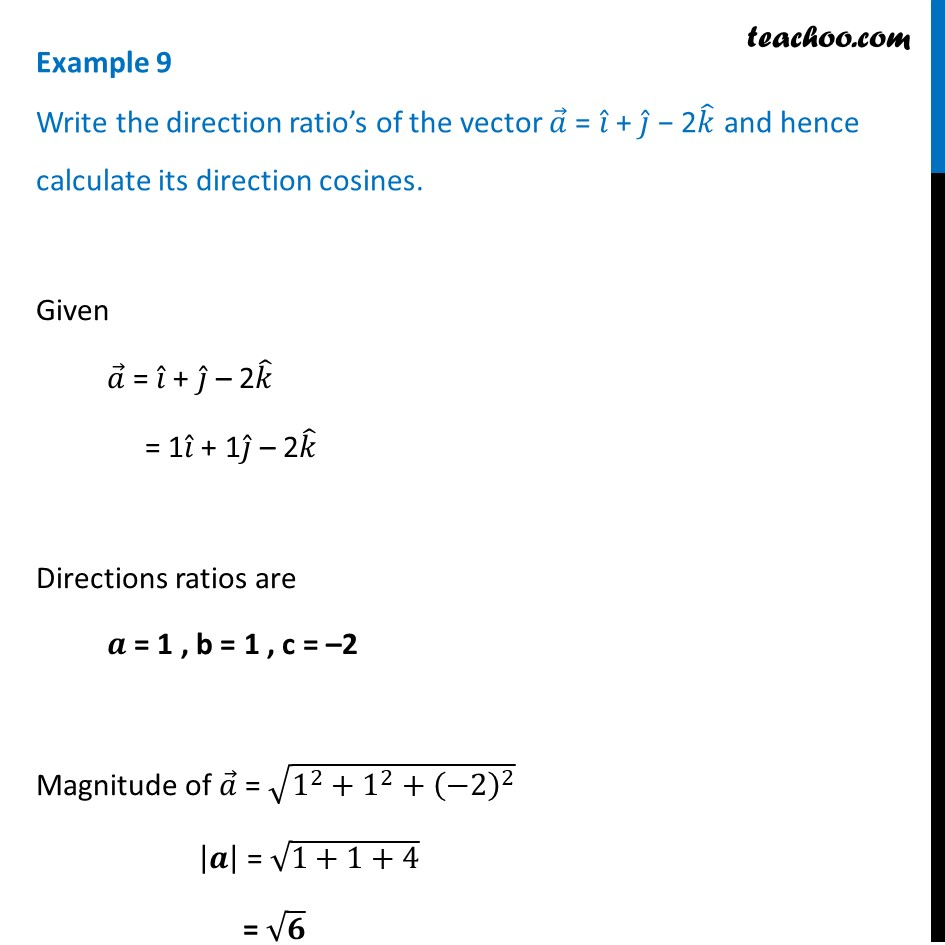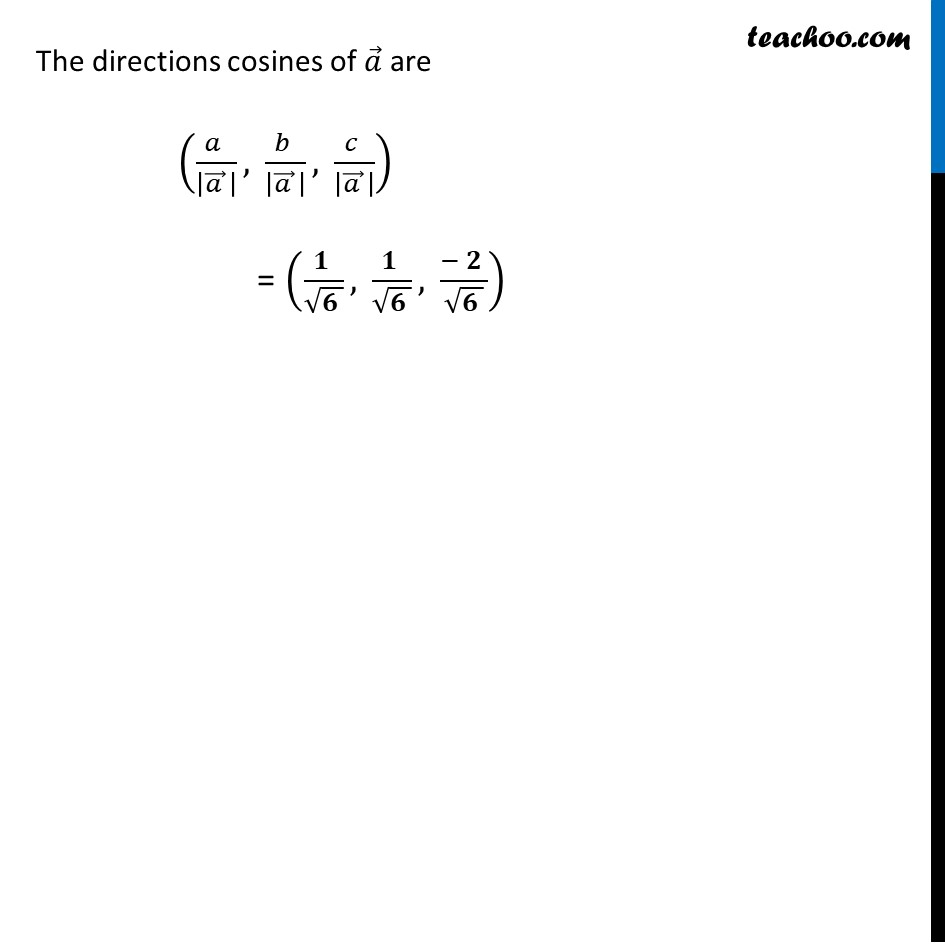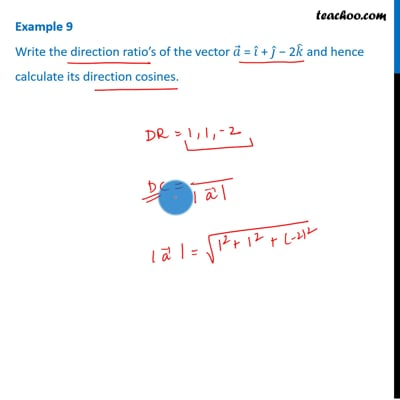Direction cosines and ratios

Chapter 10 Class 12 Vector Algebra
Concept wiseThis video is only available for Teachoo black users

Introducing your new favourite teacher - Teachoo Black, at only ₹83 per month

### Transcript

Example 9 Write the direction ratio’s of the vector 𝑎 ⃗ = 𝑖 ̂ + 𝑗 ̂ − 2𝑘 ̂ and hence calculate its direction cosines. Given 𝑎 ⃗ = 𝑖 ̂ + 𝑗 ̂ – 2𝑘 ̂ = 1𝑖 ̂ + 1𝑗 ̂ – 2𝑘 ̂ Directions ratios are 𝒂 = 1 , b = 1 , c = –2 Magnitude of 𝑎 ⃗ = √(1^2+1^2+(−2)^2 ) |𝒂| = √(1+1+4) = √𝟔 The directions cosines of 𝑎 ⃗ are (𝑎/|𝑎 ⃗ | ,𝑏/|𝑎 ⃗ | ,𝑐/|𝑎 ⃗ | ) = (𝟏/√𝟔,𝟏/√𝟔,(−𝟐)/√𝟔)